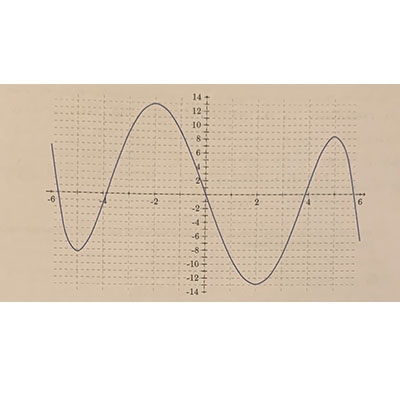# Consider the graph of the function f(x) given below. a. Consider f(x) on the closed interval...

## Question:

Consider the graph of the function {eq}f(x) {/eq} given below.

a. Consider {eq}f(x) {/eq} on the closed interval {eq}[-6,6] {/eq}. List all {eq}x {/eq} values that correspond with local maximums, and explain why the function has a local maximum for each value.

b. Consider {eq}f(x) {/eq} on the closed interval {eq}[-6,6] {/eq}. Find, if it exists, the global minimum of {eq}f(x) {/eq} on this interval explaining why it is a global minimum.

c. Is the following statement true or false, and explain your answer: "The function {eq}f(x) {/eq} has no extrema in the interval {eq}(-2,-2) {/eq}"

d. Consider {eq}f(x) {/eq} on the open interval {eq}(-2,2) {/eq}. Is there an infection point in this interval? Explain why or why not.## Functions and Critical Points:

A function can be analyzed by providing not only its domain and range, but also its maximum and minimum values and the points at which those maxima and minima occur. The curvature of the function also describes its behavior, stating whether the function is concave up or down. The points at which the nature of concavity changes are called inflection points.

a) The local maxima on the interval {eq}[-6;6] {/eq} occur at the points {eq}x = -2 {/eq} and {eq}x = 5 {/eq}

Those points are local maxima since the derivative of a function is zero at those points and the function changes its behavior from increasing to decreasing when passing through those points.

b) The global minimum of the function is at the point {eq}x = 2 {/eq}.

It is the minimum since the function has zero derivative at that point and the function switches its behavior from decreasing to increasing when passing through this point. It is a global minimum since the value of the function at that point is the smallest among all minima on the interval {eq}[-6; 6] {/eq} and the values of the function at the boundary points of the interval.

c) The statement is true.

Explanation: the derivative of a function is not zero at any point in the open interval {eq}(-2; 2) {/eq}, therefore, there is no extremal point on that interval.

d) There is an inflection point in the interval {eq}(-2; 2) {/eq}. The presence of inflection point is guaranteed because the function's second derivative changes sign from negative to positive (the function changes from being concave up to concave down). Since concavity of the function changes, there is an inflection point at which the concavity changes.